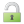# Scientific Calculator

##SasanM/fibonacci (function() { var po = document.createElement('script'); po.type = 'text/javascript'; po.async = true; po.src = 'https://apis.google.com/js/plusone.js'; var s = document.getElementsByTagName('script'); s.parentNode.insertBefore(po, s); })();

Calculate the n-th fibonacci number for given integer number n

``````fibo=function(n){
var a=0;
var b=1;
var c=0;
var i=2;
while (i <= n) -> (
c=a+b;
a=b;
b=c;
i +=1

);
c
}``````

1 Comment

• calculation of n-th Fibonacci number by given integer Number.
to execute the function type:
fibo(n) where n could be any integer number.
it is resorce dependend